# plformat

## plot text by extended C-formatstring with various justifications

parametertypeunitsdescription
leftrightfloattexthorizontal shift
updownfloattextvertical shift
formatchar*--any standard C-format
returns:void

## Description

plformat plots formatted data centered at the current position, shifted by (leftright,updown ) text unit relative to the center. Text units are the length and height of the text. Thus leftright =updown =0,5 places the lower left of the text box at the current position. format can be any valid C-language format string. As expected, the sequence \n inserts a newline, that is: a new line of text is started below the initial position. Some symbols: @ ^ _ # \ and % will be output only if they are doubled. For the latter two this is obvious as it is imposed by the C-compiler. The first four have a special significance:
@ (at_sign)
toggles between initial and alternate font (see plinit)
^ (uparrow)
toggles between normal/subscript and superscript
_ (underscore)
toggles between normal/superscript and subscript
# (hash_mark)
toggles between lower and upper ASCII table

## Examples

The following script shows how text can be justified relative to the current point:
```plinit PS plformat A4 15 15 "" ""
plset XGRID 1 plset YGRID 1
plaxes 0 0 10 6 150 100 "" "" ""
plrect 0 0 10 6
plset PENDIA 1 s=.5 a=360 r=.05
plot 7 5 UP plarc 7 5 r 0 a DOWN
plformat  s  s sin(@a+3b@)3^+a_%d_\nbottom-left 90
plot 5 3 UP plarc 5 3 r 0 a DOWN
plformat  0  0 sin(@a+3b@)3^+a_%d_\ncenter 90
plot 3 1 UP plarc 3 1 r 0 a DOWN
plformat -s -s sin(@a+3b@)3^+a_%d_\ntop-right 90
plframe 5 3

```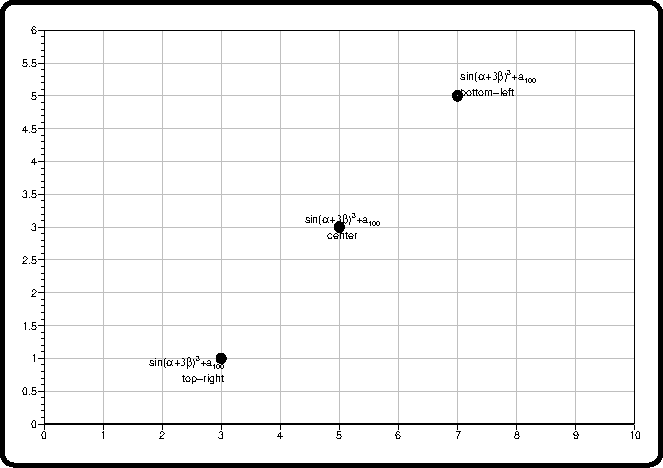The following example plots symbol tables showing the Ascii characters in a given font. Both the normal (lower Ascii) and the shifted (upper Ascii) characters are shown. Tables are shown here for the fonts Helvetica, Symbol, ZapfChancery-MediumItalic and ZapfDingbats.
```#include <stdio.h>
#include <string.h>
#include <simplot.h>
int main() {
int i,j,k,nf=4,ifont;
float x,y;
char *font[]={"H","S","ZCMI","ZD"};
char *fontname[]={"Helvetica",
"Symbol",
"ZapfChancery-MediumItalic",
"ZapfDingbats"};

for (ifont=0;ifont<nf;ifont++) {
plinit(PS,font[ifont],A4,25,269,"C",font[ifont]);
plset(HEIGHT,5);

plfont();   // switch to alt font
plformat(.5,.5,"%s - %s",font[ifont],fontname[ifont]);
plfont();   // back to normal font
plmvorgm(0,-15);

plset(HEIGHT,3);
for (i=32;i<128;i+=32) {
x=(i-32)*1.7;
for (j=0;j<32;j++) {
y=-j*6;
k=i+j;
plu(x,y);
if (strchr("@^_#",k))   // these chars must be doubled
plformat(.5,.5,"%3d (%c%c)@   %c%c  # %c%c",k,k,k,k,k,k,k);
else
plformat(.5,.5,"%3d (%c)@   %c # %c",k,k,k,k);
// # must be followed by space, else we get # instead of shifted #
}
}
plframe(5,3);
plend();
}
exit(0);
}
```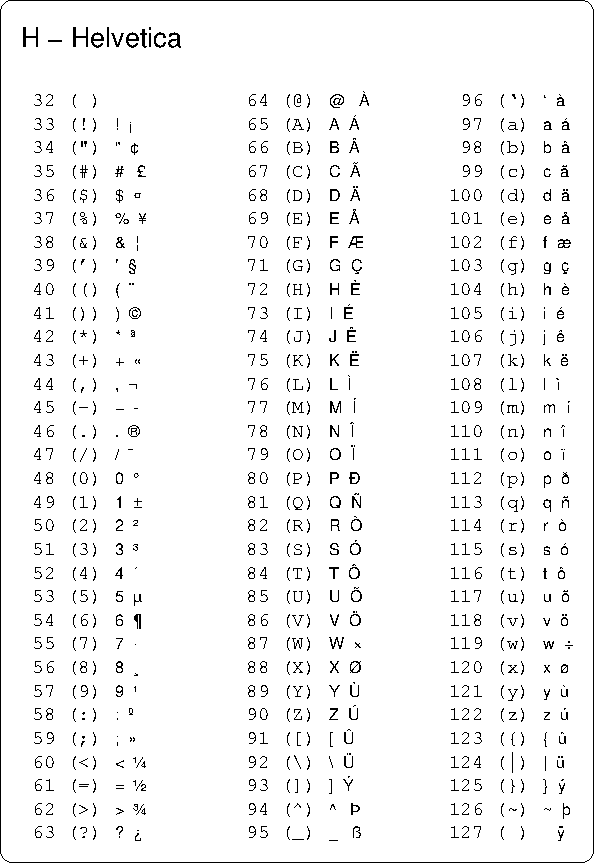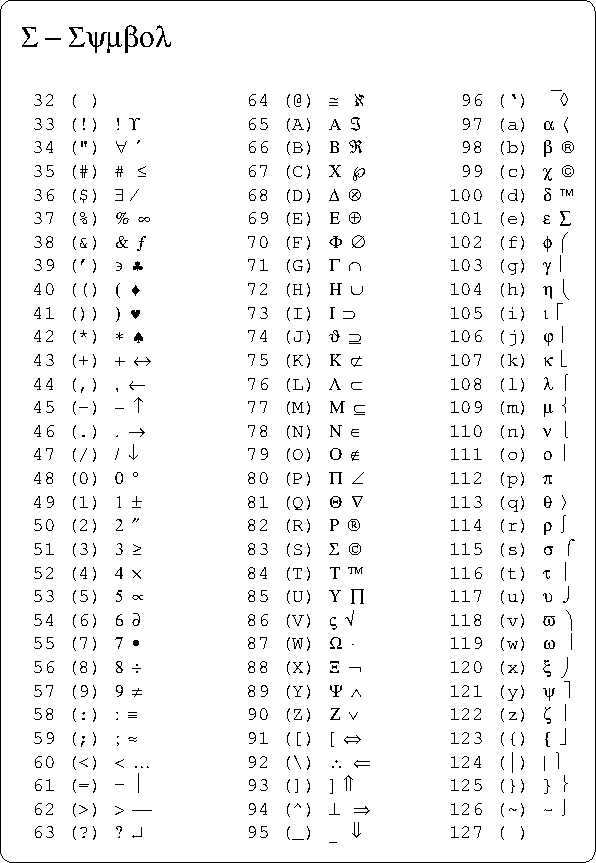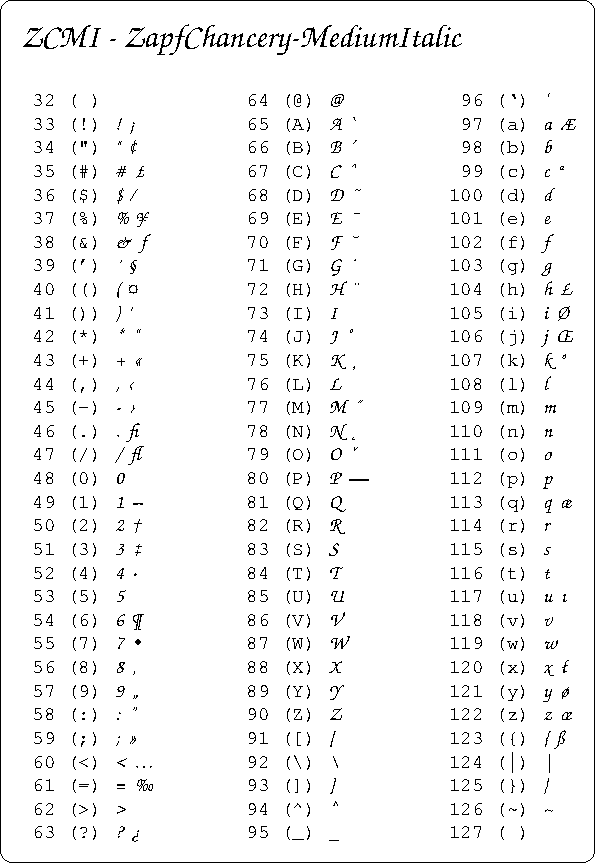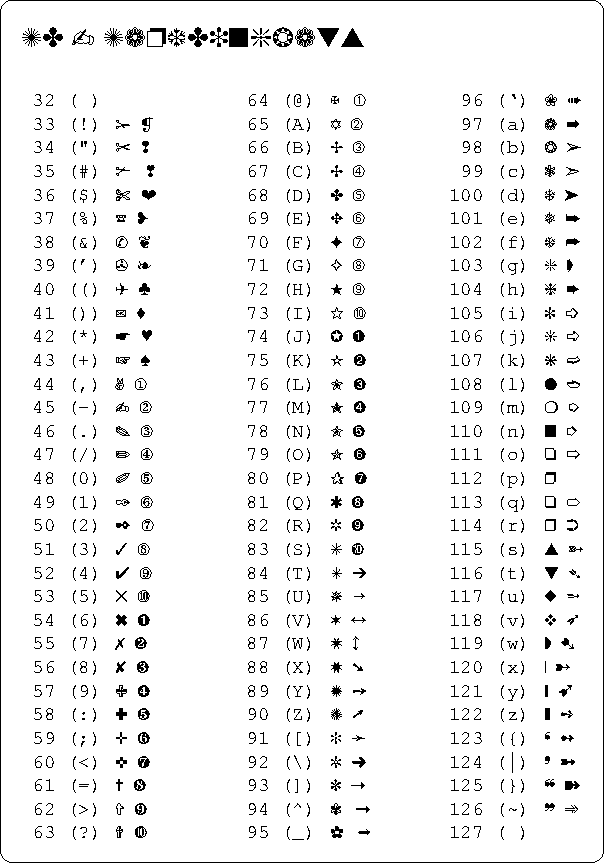plfill plclip plbox plframe plarrow plfunc plrotate pltrace plaxes plhiss plreserv plfont plloop plsmooth simplot program plpolar plpolv plot
• Home